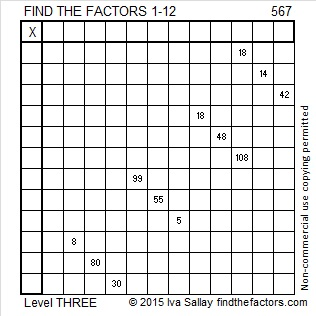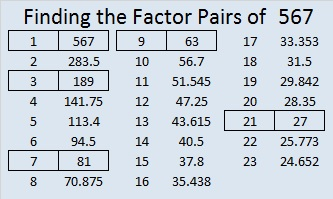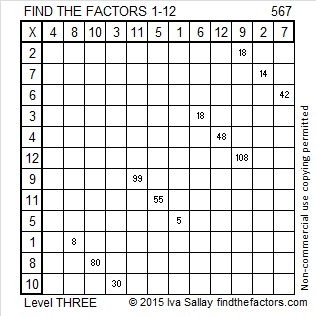# 567 and Level 3

567 is made from three consecutive numbers so it is divisible by 3. Since the middle number of those three consecutive numbers is divisible by 3, we know that 567 is also divisible by 9.

567 and its square, 321489, use all the digits 1-9 exactly once. I learned that fact from reading stetson.edu.Print the puzzles or type the solution on this excel file: 12 Factors 2015-07-27

—————————————————————————————————

• 567 is a composite number.
• Prime factorization: 567 = 3 x 3 x 3 x 3 x 7, which can be written 567 = (3^4) x 7
• The exponents in the prime factorization are 4 and 1. Adding one to each and multiplying we get (4 + 1)(1 + 1) = 5 x 2 = 10. Therefore 567 has exactly 10 factors.
• Factors of 567: 1, 3, 7, 9, 21, 27, 63, 81, 189, 567
• Factor pairs: 567 = 1 x 567, 3 x 189, 7 x 81, 9 x 63, or 21 x 27
• Taking the factor pair with the largest square number factor, we get √567 = (√81)(√7) = 9√7 ≈ 23.81176—————————————————————————————————